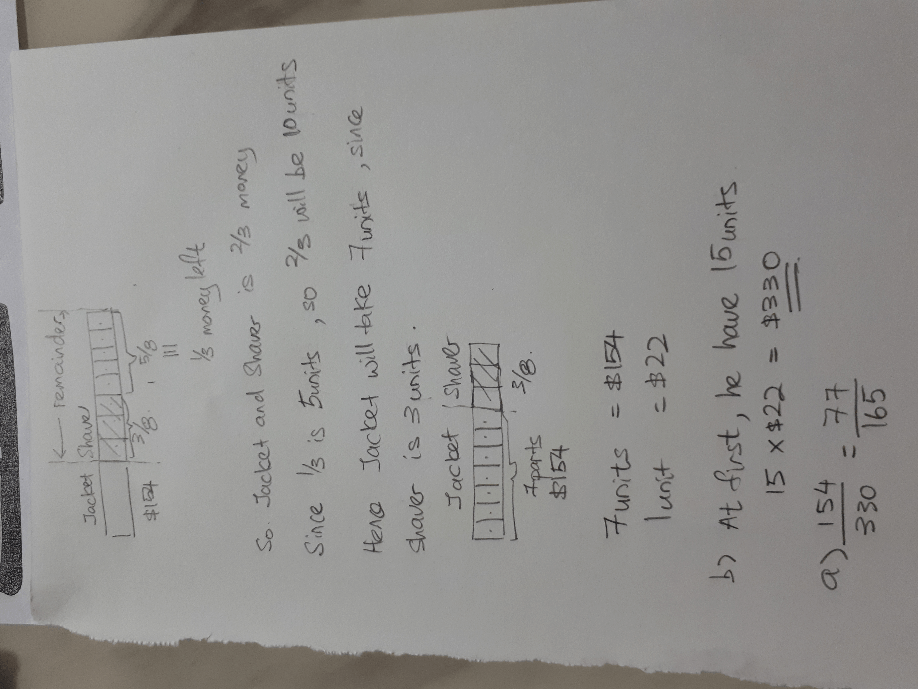# Question

Hi, we need help for this qn. Tq!

_________

Mr Suray had some money. He spent  \$154 on a jacket and 3/8 of the remainder on a shaver. He had 1/3 of his money left.

a) what fraction of the money did he spend in the jacket?

b) how much money did he have at first?

Can someone explain via both  branching and model method?

This is Part whole question.

 Jacket Shaver Left total Quantity 154 3u 5u 154 + 8u 1 3

5u/ 1 = (154 + 8u) / 3

15u = 154 + 8u

15u – 8u = 154

7u = 154

1u = 154 ÷ 7 = 22

b)

8u = 22 × 8 = 176

176 + 154 = 330

a)

154/ 330 = 77/165

Note

A Branching question has 2 fractions.

Eg 1/3 on Jacket and 3/8 of the remainder on Shaver.

This question is just a Part Whole question because it started with  a Number \$154.

0 Replies 0 Likes

a)
Branching method :
his money – 154 (jacket)
– remainder – 3/8 (shaver)
– 5/8 = 1/3 of his money

154 + 3/8 of the remainder = 2/3 of his money = 10/8 of the remainder
remainder = [154/(10 – 3)] x 8 = 176
154/(154 + 176) = 77/165

Model method :
154 + 1 unit + 1 unit + 1 unit + 1 unit + 1 unit + 1 unit + 1 unit + 1 unit

1/3 of his money = 5 units
2/3 of his money = 10 units = 154 + 3 units
1 unit = 154/(10 – 3) = 22
22 x 8 = 176
154/(154 + 176) = 77/165

(b)
154 + 176 = 330

Ans : (a) 77/165; (b) \$330.

0 Replies 0 LikesMy previous answer for a) was not right. Edited below.

I am not too sure with branching method. I did model diagram method.

Jacket cost \$154, need to find how many units for jacket.

Shaver is 3/8 remainder. Means 5/8 remainder left is 1/3 of his before money.

So jacket and shaver are 2/3 of his before money. Or 10 units from the  model. Since shaver is 3 units, so jacket is 7 units.

7u = \$154

1u = \$22

B) 15u × \$22 = \$330 he had at first.

A) fraction for jacket will be 154/330 = 77/165

0 Replies 0 Likes